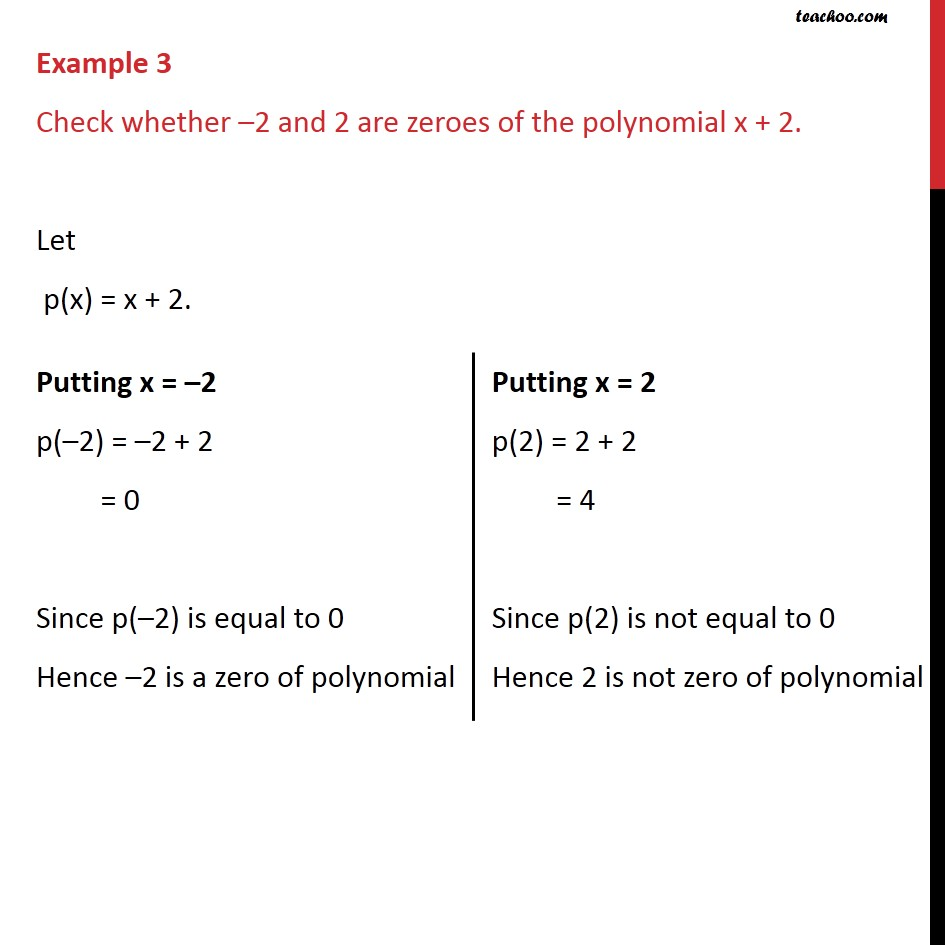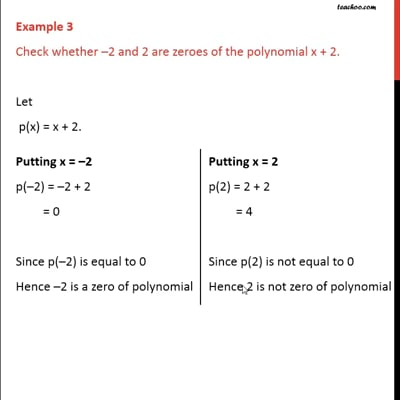Examples

Chapter 2 Class 9 Polynomials
Serial order wiseThis video is only available for Teachoo black users

Maths Crash Course - Live lectures + all videos + Real time Doubt solving!

### Transcript

Example 3 Check whether 2 and 2 are zeroes of the polynomial x + 2. Let p(x) = x + 2. Putting x = 2 p( 2) = 2 + 2 = 0 Since p( 2) is equal to 0 Hence 2 is a zero of polynomial Putting x = 2 p(2) = 2 + 2 = 4 Since p(2) is not equal to 0 Hence 2 is not zero of polynomial